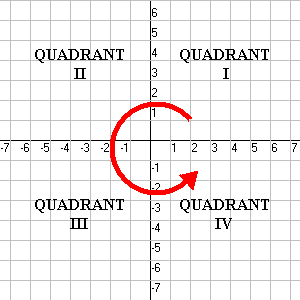The Cartesian plane is divided into four quadrants. These are numbered from $\text{I}$ through $\text{IV}$, starting with the upper right and going around counterclockwise. (For some reason everybody uses roman numerals for this).In Quadrant $\text{I}$, both the $x$– and $y$-coordinates are positive; in Quadrant $\text{II}$, the $x$-coordinate is negative, but the $y$-coordinate is positive; in Quadrant $\text{III}$ both are negative; and in Quadrant $\text{IV}$, $x$ is positive but $y$ is negative.
Points which lie on an axis (i.e., which have at least one coordinate equal to $0$) are said not to be in any quadrant. Coordinates of the form $\left(x,0\right)$ lie on the horizontal $x$-axis, and coordinates of the form $\left(0,y\right)$ lie on the vertical $y$-axis.